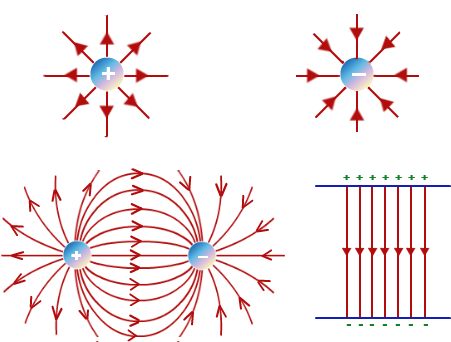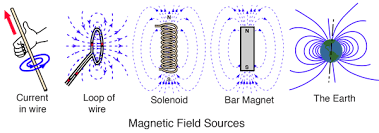# Difference between Electric Field and Magnetic Field

Key difference: Electric field is produced by stationary electric charges in the space around them whereas the magnetic field is produced by moving or flowing electric charges in the space around them.Electric and Magnetic fields are related to each other and are components of the electromagnetic force which is one of the four fundamental forces of nature. They are studied and researched in both core physics and applied physics and other branches        (See Physics Vs. Applied Physics). The main difference between electric and magnetic field lies in the way they are produced. Electric field is only because of the charge existing in the space. Higher the intensity of the charge higher is the force exerted at a constant point. Electric field force is due to voltage and it follows the inverse square law and it is a low range force. The magnitude of the electric field depends upon the voltage and the potential difference of the source electric current (see Voltage Vs. Potential Difference) and can be positive or negative depending upon what charge is producing it.

The magnetic field is produced by a flow of electric charge. Stationary charges can also produce magnetic fields but essentially the intrinsic motion of the charges themselves constitute a magnetic field. At the atomic level, the origin of magnetic force arises from the tiny electric loops. For example, in atoms, the magnetic field is produced by the electron loops (i.e. the electrons revolving in orbits around the atomic nucleus theoretically considered as a flow of current). Magnetic field force is related to an intrinsic quantum property called spin that gives subatomic particles a magnetic dipole moment. Electric fields give rise to the magnetic field and that is apparent in magnetized metals. Artificial permanent magnets are produced by aligning all the electron loops in the metal in one direction i.e. a common orientation. This process is known as magnetization and that is possible only with ferrous metals (see Ferrous Vs. Non-ferrous metals). The net magnetic moment of the magnet is equal to the sum of the individual magnetic moments of all the oriented atomic loops. Magnetic fields are related to the changes in the motion and orientations of the electric charges. Electrical appliances and electronics have functions mainly depending upon electric and magnetic fields. See the (Electricals Vs. Electronics).Electric field force is proportional to the amount of charge and is inversely proportional to the square of the distance between the test point and the charge. one difference between electric and magnetic field is that the inverse square law for the electric intensity of the electric field applies only for stationary charges and not for moving charges. The magnetic field is proportional to the amount of moving charge and the speed of the moving charge. The electric field is measured as the force per unit charge experienced by a stationary point charge at a given position in the electric field whereas the magnetic field is measured by the force it exerts on other magnetic particles and charges in motion in the field. Electric field moves in the direction perpendicular to the magnetic field in an electromagnetic field whereas magnetic field moves in the direction perpendicular to the electric field in the electromagnetic field. Magnetism is mainly utilized in induction motors and other gadgets where dynamic parts are involved. See the Synchronous motors Vs. Induction motors).

One key difference between an electric and magnetic field that is a popular topic of discussion is that an electric monopole (single charge) exists but a magnetic monopole (either N-pole or S- pole) does not exist in nature. If we break a magnet into two or more pieces each one of them develops a north and a south pole instantly. This is a hot topic in physics research as it is theoretically possible to have a magnetic monopole but physicists are still wondering why magnetic monopoles do not exist.

 Difference between electric and magnetic field Electric field Magnetic field Nature Created around an electric charge Created around moving electric charge and magnets Units Newton per coulomb, volts per meter Gauss or Tesla Force Proportional to the electric charge Proportional to charge and speed of electric charge Movement In Electromagnetic field Perpendicular to the magnetic field Perpendicular to the electric field Electromagnetic Field Generates VARS (Capacitive) Absorbs VARS (Inductive) Pole Monopole or Dipole Dipole

Also see: Current Vs. Voltage

Image credits: www.tutorvista.comhttp://hyperphysics.phy-astr.gsu.edu